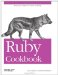# Doing Date Arithmetic

Problem

You want to find how much time has elapsed between two dates, or add a number to a date to get an earlier or later date.

Solution

Adding or subtracting a Time object and a number adds or subtracts that number of seconds. Adding or subtracting a Date object and a number adds or subtracts that number of days:

```	require 'date'
y2k = Time.gm(2000, 1, 1) # => Sat Jan 01 00:00:00 UTC 2000
y2k + 1 # => Sat Jan 01 00:00:01 UTC 2000
y2k - 1 # => Fri Dec 31 23:59:59 UTC 1999
y2k + (60 * 60 * 24 * 365) # => Sun Dec 31 00:00:00 UTC 2000

y2k_dt = DateTime.new(2000, 1, 1)
(y2k_dt + 1).to_s # => "2000-01-02T00:00:00Z"
(y2k_dt - 1).to_s # => "1999-12-31T00:00:00Z"
(y2k_dt + 0.5).to_s # => "2000-01-01T12:00:00Z"
(y2k_dt + 365).to_s # => "2000-12-31T00:00:00Z"
```

Subtracting one Time from another gives the interval between the dates, in seconds. Subtracting one Date from another gives the interval in days:

```	day_one = Time.gm(1999, 12, 31)
day_two = Time.gm(2000, 1, 1)
day_two - day_one # => 86400.0
day_one - day_two # => -86400.0

day_one = DateTime.new(1999, 12, 31)
day_two = DateTime.new(2000, 1, 1)
day_two - day_one # => Rational(1, 1)
day_one - day_two # => Rational(-1, 1)

# Compare times from now and 10 seconds in the future.
before_time = Time.now
before_datetime = DateTime.now
sleep(10)
Time.now - before_time # => 10.003414
DateTime.now - before_datetime # => Rational(5001557, 43200000000)
```

The activesupport gem, a prerequisite of Ruby on Rails, defines many useful functions on Numeric and Time for navigating through time:

 So does the Facets More library.

```	require 'rubygems'
require 'active_support'

10.days.ago # => Wed Mar 08 19:54:17 EST 2006
1.month.from_now # => Mon Apr 17 20:54:17 EDT 2006
2.weeks.since(Time.local(2006, 1, 1)) # => Sun Jan 15 00:00:00 EST 2006

y2k - 1.day # => Fri Dec 31 00:00:00 UTC 1999
y2k + 6.3.years # => Thu Apr 20 01:48:00 UTC 2006
6.3.years.since y2k # => Thu Apr 20 01:48:00 UTC 2006
```

Discussion

Ruby's date arithmetic takes advantage of the fact that Ruby's time objects are stored internally as numbers. Additions to dates and differences between dates are handled by adding to and subtracting the underlying numbers. This is why adding 1 to a Time adds one second and adding 1 to a DateTime adds one day: a Time is stored as a number of seconds since a time zero, and a Date or DateTime is stored as a number of days since a (different) time zero.

Not every arithmetic operation makes sense for dates: you could "multiply two dates" by multiplying the underlying numbers, but that would have no meaning in terms of real time, so Ruby doesn't define those operators. Once a number takes on aspects of the real world, there are limitations to what you can legitimately do to that number.

Here's a shortcut for adding or subtracting big chunks of time: using the right-or left-shift operators on a Date or DateTime object will add or subtract a certain number number of months from the date.

```	(y2k_dt >> 1).to_s # => "2000-02-01T00:00:00Z"
(y2k_dt << 1).to_s # => "1999-12-01T00:00:00Z"
```

You can get similar behavior with activesupport's Numeric#month method, but that method assumes that a "month" is 30 days long, instead of dealing with the lengths of specific months:

```	y2k + 1.month # => Mon Jan 31 00:00:00 UTC 2000
y2k - 1.month # => Thu Dec 02 00:00:00 UTC 1999
```

By contrast, if you end up in a month that doesn't have enough days (for instance, you start on the 31st and then shift to a month that only has 30 days), the standard library will use the last day of the new month:

```	# Thirty days hath September…
halloween = Date.new(2000, 10, 31)
(halloween << 1).to_s # => "2000-09-30"
(halloween >> 1).to_s # => "2000-11-30"
(halloween >> 2).to_s # => "2000-12-31"

leap_year_day = Date.new(1996, 2, 29)
(leap_year_day << 1).to_s # => "1996-01-29"
(leap_year_day >> 1).to_s # => "1996-03-29"
(leap_year_day >> 12).to_s # => "1997-02-28"
(leap_year_day << 12 * 4).to_s # => "1992-02-29"
```

• Recipe 3.4, "Iterating Over Dates"
• Recipe 3.6, "Counting the Days Since an Arbitrary Date"
• The RDoc for Rails' ActiveSupport::CoreExtensions::Numeric::Time module (http://api.rubyonrails.com/classes/ActiveSupport/CoreExtensions/Numeric/Time.html)Ruby Cookbook (Cookbooks (OReilly))
ISBN: 0596523696
EAN: 2147483647
Year: N/A
Pages: 399